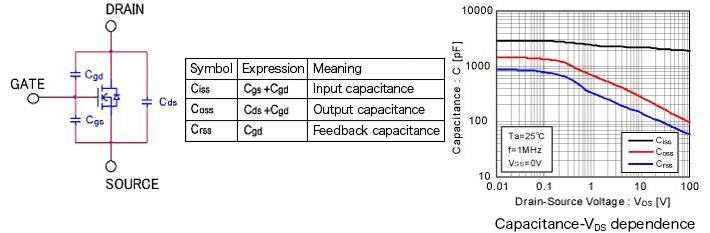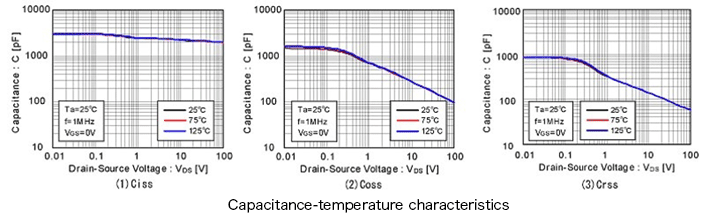Technical Information Site of Power Supply Design

2017.03.09 Si Power Device

# What are MOSFETs? - MOSFET Parasitic Capacitance and Its Temperature Characteristic

Si Transistors

In continuation of the previous discussion of Si transistor types, features and basic characteristics, we here provide an additional explanation of the characteristics of Si MOSFETs that are at present widely used as power switches.

MOSFET Parasitic Capacitance

Due to their structure, MOSFETs have a parasitic capacitance, as indicated in the diagram below. The diagram below is for an example of an N-channel MOSFET, but the situation is much the same for P-channel devices. In the power MOSFETs we are here considering that handle large amounts of power, the parasitic capacitance must be regarded as a parameter that limits the usage frequency and switching speed.

The drain and source of a MOSFET are insulated from the gate by the gate oxide film. A PN junction is formed between the drain and source with substrate intervening, and a parasitic ("body") diode is present.

The gate-source capacitance Cgs and gate-drain capacitance Cgd in the diagram below are determined by the capacitance of the gate oxide film. The drain-source capacitance Cds is the junction capacitance of the parasitic diode.The three parameters Ciss, Coss, Crss appearing on MOSFET data sheets in general relate to these parasitic capacitances. On data sheets which provide separate descriptions of static characteristics and dynamic characteristics, these are classified as dynamic characteristics. These are important parameters affecting switching performance.

Ciss is the input capacitance, and is the capacitance obtained by totaling the gate-source capacitance Cgs and the gate-drain capacitance Cgd; it is the capacitance of the MOSFET as a whole, as seen from the input. This capacitance must be driven (charged) in order to cause the MOSFET to operate, and so is a parameter of importance when studying the drivability of an input device or input losses. Qg is the amount of charge necessary to drive (charge) Ciss.

Coss is the output capacitance, obtained by adding the drain-source capacitance Cds and the gate-drain capacitance Cgs, and is the total capacitance on the output side. If Coss is large, a current arising due to Coss flows at the output even when the gate is turned off, and time is required for the output to turn off completely.

Crss is the gate-drain capacitance Cgd itself, and is called the feedback capacitance or the reverse transfer capacitance. If Crss is large, the rise in drain current is delayed even after the gate is turned on, and the fall in current is delayed after the gate is turned off. In other words, this parameter greatly affects switching speed. Qgd is the charge amount necessary to drive (charge) Crss.

These capacitances exhibit a dependence on the drain-source voltage VDS. As indicated in the graph, there is a tendency for capacitance values to be reduced as VDS is increased.

Temperature Characteristic of MOSFET Parasitic Capacitances

Ciss, Coss and Crss change hardly at all with temperature. Hence it can be said that switching characteristics are hardly affected at all by temperature changes. Actual measurement examples are shown below.Here we have explained parasitic capacitances, which are dynamic characteristics of MOSFETs. Next time, we will discuss device switching.

#### Key Points:

・MOSFETs have parasitic capacitances, which are important parameters that have an effect on switching characteristics.

・Parasitic capacitances change hardly at all with temperature, and so temperature changes exert almost no effect on switching characteristics.